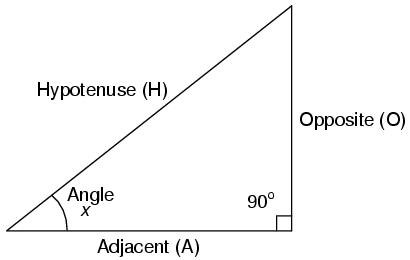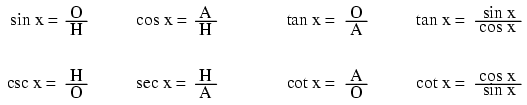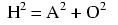# Learning Electronics

Learn to build electronic circuits

# Right triangle trigonometryA right triangle is defined as having one angle precisely equal to 90o (a right angle).

### Trigonometric identitiesH is the Hypotenuse, always being opposite the right angle. Relative to angle x, O is the Opposite and A is the Adjacent.

"Arc" functions such as "arcsin", "arccos", and "arctan" are the complements of normal trigonometric functions. These functions return an angle for a ratio input. For example, if the tangent of 45o is equal to 1, then the "arctangent" (arctan) of 1 is 45o. "Arc" functions are useful for finding angles in a right triangle if the side lengths are known.

### The Pythagorean theorem